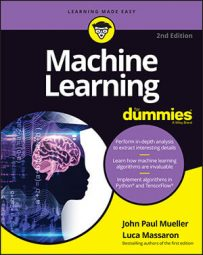##### Machine Learning For DummiesFacial recognition is important for machine learning. The capability to recognize a face in the crowd has become an essential tool for many professions. For example, both the military and law enforcement rely on it heavily. Of course, facial recognition has uses for security and other needs as well.

This example looks at facial recognition in a more general sense. You may have wondered how social networks manage to tag images with the appropriate label or name. The following example demonstrates how to perform this task by creating the right features using eigenfaces.

Eigenfaces is an approach to facial recognition based on the overall appearance of a face, not on its particular details. By means of technique that can intercept and reshape the variance present in the image, the reshaped information is treated like the DNA of a face, thus allowing recovery of similar faces (because they have similar variances) in a host of facial images.

It’s a less effective technique than extracting features from the details of an image, yet it works, and you can implement it quickly on your computer. This approach demonstrates how machine learning can operate with raw pixels, but it’s more effective when you change image data into another kind of data. You can learn more about eigenfaces or by trying the tutorial that explores variance decompositions in Scikit-learn.

In this example, you use eigenfaces to associate images present in a training set with those in a test set, initially using some simple statistical measures.

`import numpy as np`

`from sklearn.datasets import fetch_olivetti_faces`

`dataset = fetch_olivetti_faces(shuffle=True,`

`random_state=101)`

`train_faces = dataset.data[:350,:]`

`test_faces = dataset.data[350:,:]`

`train_answers = dataset.target[:350]`

`test_answers = dataset.target[350:]`

The example begins by using the Olivetti faces dataset, a public domain set of images readily available from Scikit-learn. For this experiment, the code divides the set of labeled images into a training and a test set. You need to pretend that you know the labels of the training set but don’t know anything from the test set. As a result, you want to associate images from the test set to the most similar image from the training set.

`print (dataset.DESCR)`

The Olivetti dataset consists of 400 photos taken from 40 people (so there are 10 photos of each person). Even though the photos represent the same person, each photo has been taken at different times during the day, with different light and facial expressions or details (for example, with glasses and without). The images are 64 x 64 pixels, so unfolding all the pixels into features creates a dataset made of 400 cases and 4,096 variables. It seems like a high number of features, and actually, it is. Using `RandomizedPCA`, you can reduce them to a smaller and more manageable number.

`from sklearn.decomposition import RandomizedPCA`

`n_components = 25`

`Rpca = RandomizedPCA(n_components=n_components,`

`whiten=True,`

`random_state=101).fit(train_faces)`

`print ('Explained variance by %i components: %0.3f' %`

`(n_components,`

`np.sum(Rpca.explained_variance_ratio_)))`

`compressed_train_faces = Rpca.transform(train_faces)`

`compressed_test_faces = Rpca.transform(test_faces)`

` `

`Explained variance by 25 components: 0.794`

The `RandomizedPCA` class is an approximate PCA version, which works better when the dataset is large (has many rows and variables). The decomposition creates 25 new variables (n_components parameter) and whitening (`whiten=True`), removing some constant noise (created by textual and photo granularity) and irrelevant information from images in a different way from the filters just discussed. The resulting decomposition uses 25 components, which is about 80 percent of the information held in 4,096 features.

`import matplotlib.pyplot as plt`

`photo = 17 # This is the photo in the test set`

`print ('We are looking for face id=%i'`

`% test_answers[photo])`

`plt.subplot(1, 2, 1)`

`plt.axis('off')`

`plt.title('Unknown face '+str(photo)+' in test set')`

`plt.imshow(test_faces[photo].reshape(64,64),`

`cmap=plt.cm.gray, interpolation='nearest')`

`plt.show()`

Here is the chosen photo, subject number 34, from the test set.

After the decomposition of the test set, the example takes the data relative only to photo 17 and subtracts it from the decomposition of the training set. Now the training set is made of differences with respect to the example photo. The code squares them (to remove negative values) and sums them by row, which results in a series of summed errors. The most similar photos are the ones with the least squared errors, that is, the ones whose differences are the least.

`#Just the vector of value components of our photo`

`mask = compressed_test_faces[photo,]`

`squared_errors = np.sum((compressed_train_faces –`

`mask)**2,axis=1)`

`minimum_error_face = np.argmin(squared_errors)`

`most_resembling = list(np.where(squared_errors < 20))`

`print ('Best resembling face in train test: %i' %`

`train_answers[minimum_error_face])`

` `

`Best resembling face in train test: 34`

As it did before, the code can now display photo 17, which is the photo that best resembles images from the train set.

`import matplotlib.pyplot as plt`

`plt.subplot(2, 2, 1)`

`plt.axis('off')`

`plt.title('Unknown face '+str(photo)+' in test set')`

`plt.imshow(test_faces[photo].reshape(64,64),`

`cmap=plt.cm.gray, interpolation='nearest')`

`for k,m in enumerate(most_resembling[:3]):`

`plt.subplot(2, 2, 2+k)`

`plt.title('Match in train set no. '+str(m))`

`plt.axis('off')`

`plt.imshow(train_faces[m].reshape(64,64),`

`cmap=plt.cm.gray, interpolation='nearest')`

`plt.show()`

Even though the most similar photo is similar (it’s just scaled slightly differently), the other two photos are quite different. However, even though those photos don’t match the test image as well, they really do show the same person as in photo 17.